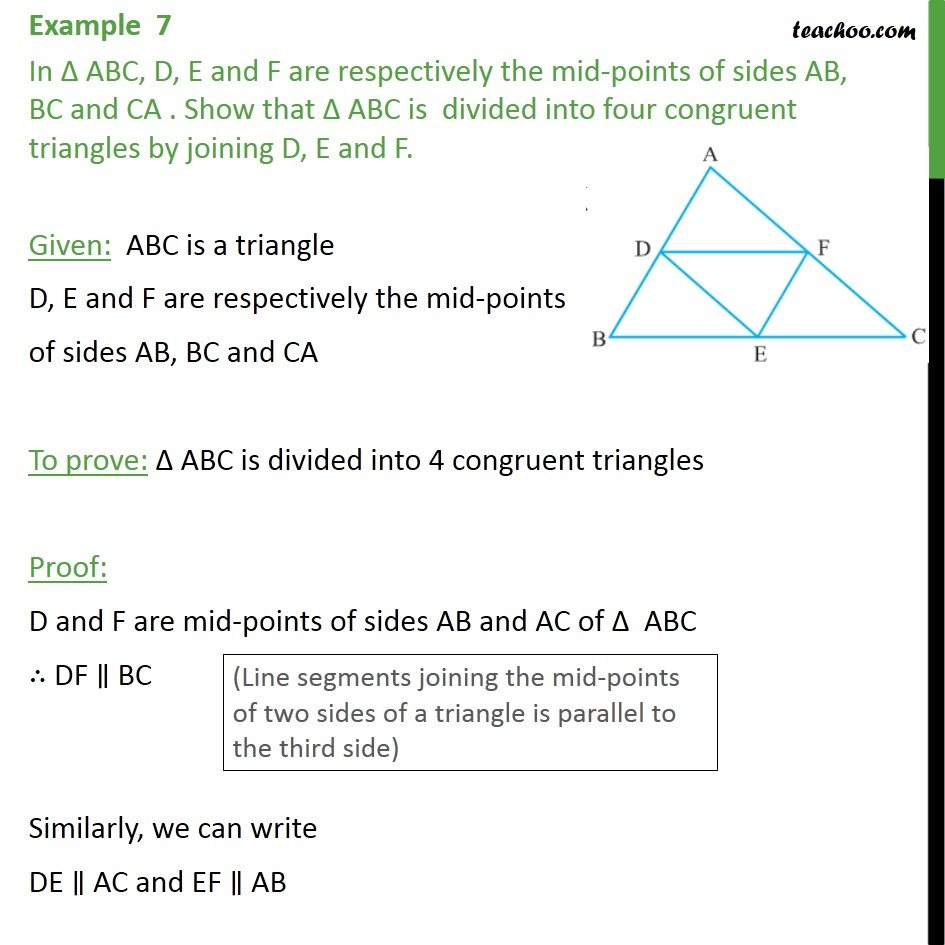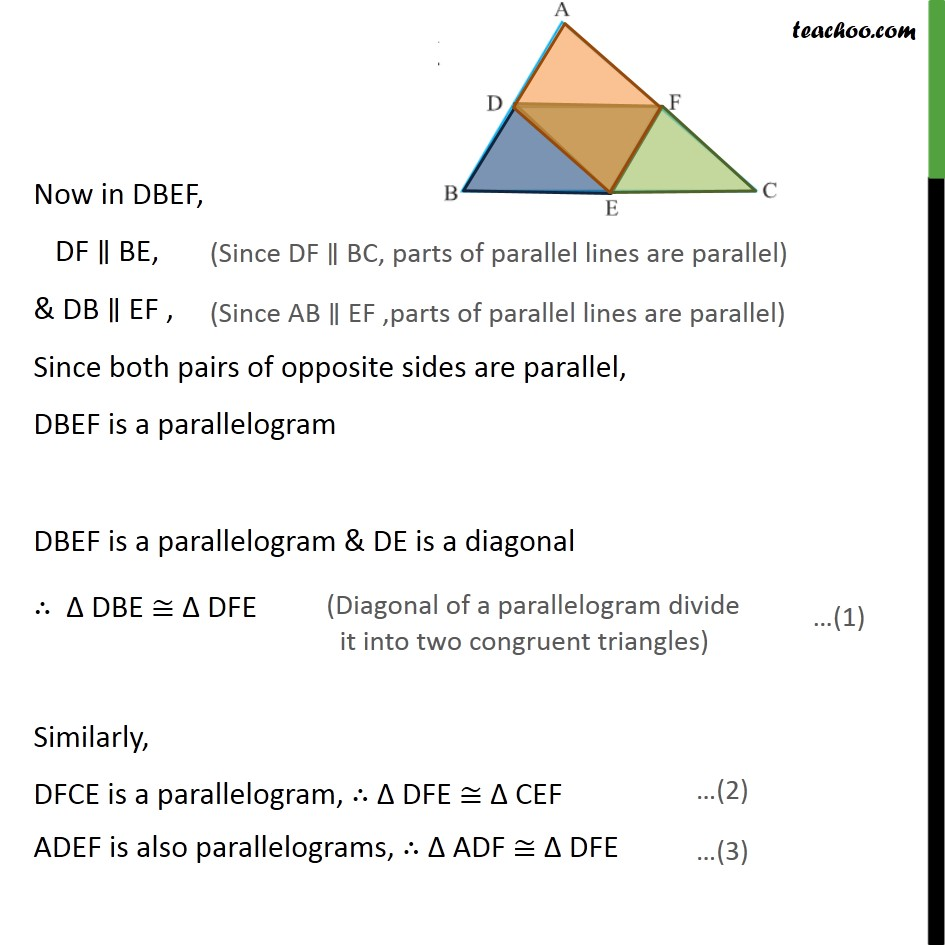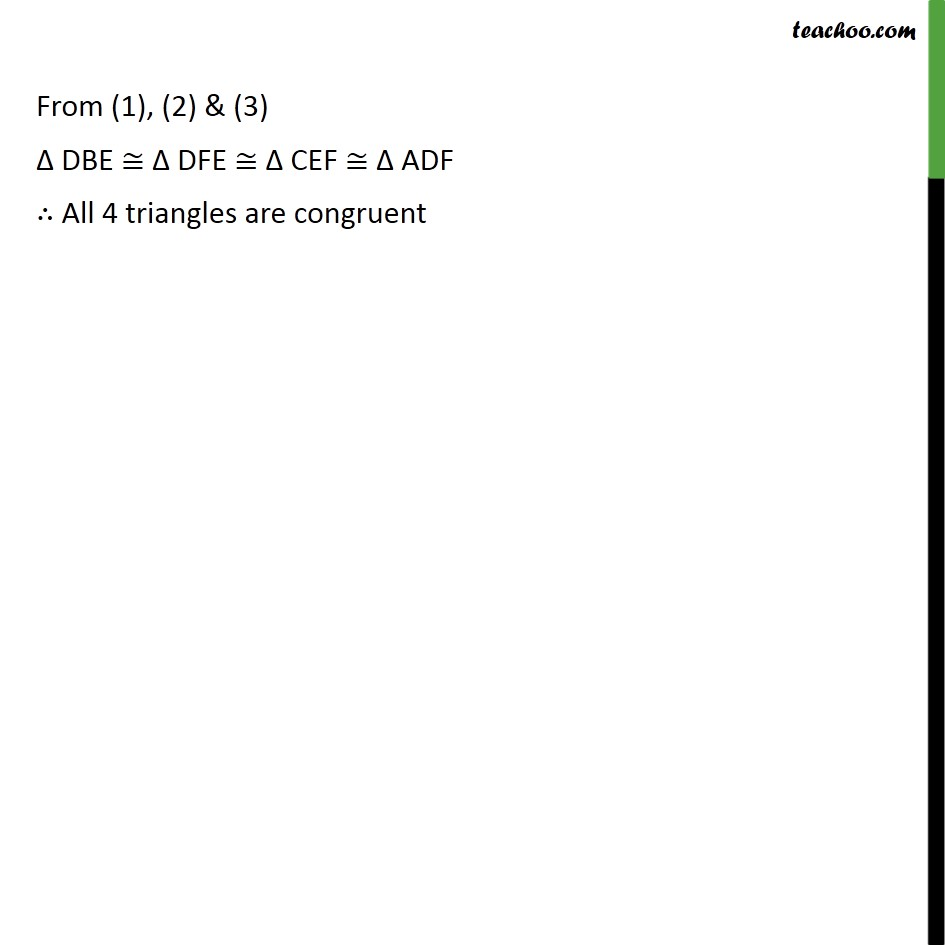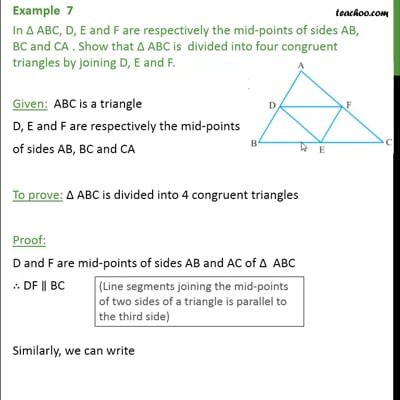Examples

Chapter 8 Class 9 Quadrilaterals
Serial order wiseThis video is only available for Teachoo black users

Solve all your doubts with Teachoo Black (new monthly pack available now!)

### Transcript

Example 7 In Δ ABC, D, E and F are respectively the mid-points of sides AB, BC and CA . Show that Δ ABC is divided into four congruent triangles by joining D, E and F. Given: ABC is a triangle D, E and F are respectively the mid-points of sides AB, BC and CA To prove: ∆ ABC is divided into 4 congruent triangles Proof: D and F are mid-points of sides AB and AC of ∆ ABC ∴ DF ∥ BC Similarly, we can write DE ∥ AC and EF ∥ AB Now in DBEF, DF ∥ BE, & DB ∥ EF , Since both pairs of opposite sides are parallel, DBEF is a parallelogram DBEF is a parallelogram & DE is a diagonal ∴ Δ DBE ≅ Δ DFE Similarly, DFCE is a parallelogram, ∴ Δ DFE ≅ Δ CEF ADEF is also parallelograms, ∴ Δ ADF ≅ Δ DFE From (1), (2) & (3) Δ DBE ≅ Δ DFE ≅ Δ CEF ≅ Δ ADF ∴ All 4 triangles are congruent